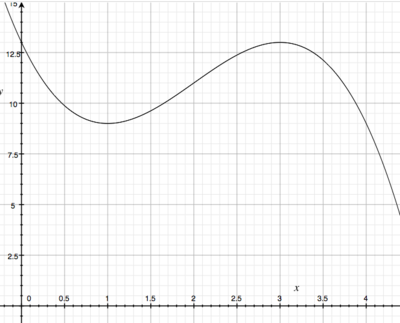# Find the coordinates of points where the graph of f(x) has horizontal tangents. As a check, graph...

## Question:

Find the coordinates of points where the graph of f(x) has horizontal tangents. As a check, graph f(x) and see whether the points you found look as though they have horizontal tangents.

{eq}f(x) = -x^3 + 6x^2 - 9x + 13 {/eq}

## Horizontal Tangent Line

The slope of a tangent line to a function is the value of the derivative at a point. Since the slope of a horizontal line is zero, a horizontal tangent line occurs when the derivative is equal to zero. These points are also called critical points.

In order to find where this function has horizontal tangent lines, we need to first differentiate this function. This is a polynomial, which can be differentiated by applying the power rule.

{eq}f'(x) = -3x^2 + 12x - 9 {/eq}

A horizontal line has a slope of zero, and since the slope of a tangent line comes from the derivative, we can find where the function has horizontal tangent lines by finding when the derivative equals zero.

{eq}-3x^2 + 12x - 9 = 0\\ -3(x^2 - 4x + 3) = 0\\ -3(x-3)(x-1) = 0\\ x = 3\\ x = 1 {/eq}

Let's graph this function to show that these are the two points where this function has a horizontal tangent line.